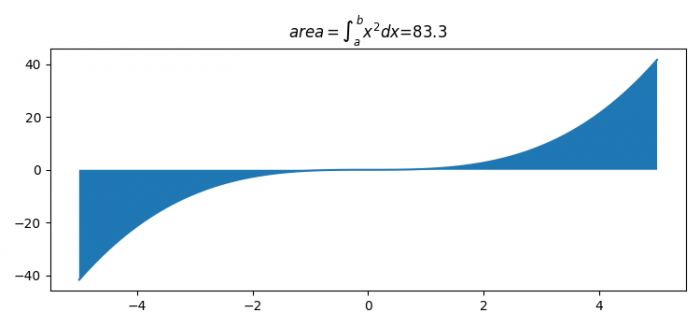# How can I format a float using matplotlib's LaTeX formatter?

To format a float using matplotlib's LaTeX formatter, we can take the following steps −

## Steps

• Set the figure size and adjust the padding between and around the subplots.

• Create x and y data points using numpy.

• Plot the x and y data points using plot() method.

• Fill the area between the curve.

• Set the title of the figure with LaTeX representation.

• To display the figure, use show() method.

## Example

import numpy as np
from matplotlib import pyplot as plt

# Set the figures size
plt.rcParams["figure.figsize"] = [7.50, 3.50]
plt.rcParams["figure.autolayout"] = True

# x and y data points
x = np.linspace(-5, 5, 100)
y = x**3/3

# Plot the data points
plt.plot(x, y)

# Fill the area between the curve
plt.fill_between(x, y)

# LaTex representation
plt.title("$area=\int_a^b{x^2dx}$=83.3")

# Display the plot
plt.show()

## Output

It will produce the following output −